# Test: F Block Elements - II (Actinides)

## 25 Questions MCQ Test Chemistry Class 12 | Test: F Block Elements - II (Actinides)

Description
This mock test of Test: F Block Elements - II (Actinides) for Class 12 helps you for every Class 12 entrance exam. This contains 25 Multiple Choice Questions for Class 12 Test: F Block Elements - II (Actinides) (mcq) to study with solutions a complete question bank. The solved questions answers in this Test: F Block Elements - II (Actinides) quiz give you a good mix of easy questions and tough questions. Class 12 students definitely take this Test: F Block Elements - II (Actinides) exercise for a better result in the exam. You can find other Test: F Block Elements - II (Actinides) extra questions, long questions & short questions for Class 12 on EduRev as well by searching above.
QUESTION: 1

### Only One Option Correct Type Direction (Q. Nos. 1-10) This section contains 10 multiple choice questions. Each question has four choices (a), (b), (c) and (d), out of which ONLY ONE is correct. Q.  Which one of the following is an electronic configuration of thorium?

Solution:

Thorium (Z = 90) have [Rn] 5f06d27s2 configuration.

QUESTION: 2

### Which of the following is general electronic configuration of actinides?

Solution:

General EC = [Rn] 5f1-146d0-17s2.

QUESTION: 3

### The actinoids Exhibit more member of oxidation states in general than the lanthanoids. This is because

Solution:

The wide range of oxidation state of actinoids is due to the fact that the 5f, 6d and 7s energy levels are of comparable energies and all these three subshells can participate in comparison to lanthanoid. The 5f orbitals extend farther from the nucleus than the 4f orbitals.

QUESTION: 4

Identify the incorrect statement among the following.

Solution:

4f electrons have greater shielding effect but 5f electrons have poor shielding effect.

QUESTION: 5

In which 5f subshell is half-filled?

Solution:

Americium (2 = 95) and curium (Cm, Z = 96) have [Rn] 5f76d07s2 and [Rn] 5f76d17s2 configuration, respectively.

QUESTION: 6

Actinides

Solution:

Actinides are highly electropositive and reactive metals.

QUESTION: 7

Which of the following is not an actinide?

Solution:

Actinoids are from thorium (Z = 90)to Lawrencium (Z = 103) in the Periodic table So, rutherfordium (Z = 104) is not an actinoid.

QUESTION: 8

The compound used in enrichment of uranium for nuclear power plant is

Solution:

UF6 is used in enrichment of uranium for nuclear power plant.

QUESTION: 9

Which one of the following shows oxidation state upto + 7?

Solution:

Plutonium (Z = 94) have [Rn] 5f66d07s2 configuration and show oxidation state upto +7.

QUESTION: 10

The general electronic configuration of actinides is (n - 2) f1-14 (n - 1) d0-1 ns2.

Which one of the following actinides has one electron in 6d-orbital?

Solution:

Cm(Z = 96)have [Rn] 5f76d17s2,
Lr (Z = 103) have [Rn] 5f146d17s2
Np (Z = 93) have [Rn] 5f46d17s2

*Multiple options can be correct
QUESTION: 11

Direction (Q. Nos. 11-15) This section contains 5 multiple choice questions. Each question has four choices (a), (b), (c) and (d), out of which ONE or MORE THAN ONE are correct.

Q.

Commonly used nuclear fuels are

Solution:

Uranium is used in the production of nuclear energy by the process of nuclear fission.
Plutonium is fissionable material and is used for fueling atomic reactors.

*Multiple options can be correct
QUESTION: 12

Which are the correct statements?

Solution:

Actinoids are radioactive elements and uranium is the heaviest natural element.

*Multiple options can be correct
QUESTION: 13

Pick up the incorrect statements.

Solution:

Correct statements are oxides and hydroxides of actinides are more basic than lanthanoids and actinoids have greater tendency to form complexes in comparison to lanthanoids.

*Multiple options can be correct
QUESTION: 14

Which species have half-filled 5f subshell?

Solution:

Am (Z = 95) have [Rn] 5f76d07s2 Am2+ have [Rn] 5f76d0, Cm (Z = 96) have [Rn] 5f76d17s2
NP (Z = 93) have [Rn] 5f46d17s2

*Multiple options can be correct
QUESTION: 15

Which are correct regarding actinides?

Solution:

Alkalis have no action with actinoids metals.

QUESTION: 16

Comprehension Type

Direction (Q. Nos. 16 and 17) This section contains a paragraph, describing theory, experiments, data, etc. Two questions related to the paragraph have been given. Each question has only one correct answer among the four given options (a), (b), (c) and (d).

Passage

Rutherford and Soddy found that every uranium ore that they examined contained minute quantities of radium and an appreciable amount of non-radioactive lead. From this, they concluded that uranium is the starting substance in a series of radioactive transformations which pass through radium and ultimately ends with stable lead. These transformations are accompanied by spontaneous emissions of alpha and beta particles resulting in the formation of new atoms. All the nuclei from the initial element to the final stable element constitute a series called disintegration series. Mass number changes by 4 units per each alpha particle emission.
There are four disintegration series. They are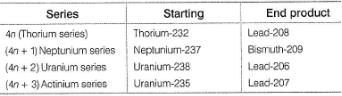Q.

The number of alpha and beta particles emitted in the nuclear reaction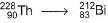are

Solution:

Number of alpha particles emitted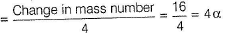Number of beta particles liberated
= Double the alpha particles - change in atomic number
= 8 - 7 = 1

QUESTION: 17

Rutherford and Soddy found that every uranium ore that they examined contained minute quantities of radium and an appreciable amount of non-radioactive lead. From this, they concluded that uranium is the starting substance in a series of radioactive transformations which pass through radium and ultimately ends with stable lead. These transformations are accompanied by spontaneous emissions of alpha and beta particles resulting in the formation of new atoms. All the nuclei from the initial element to the final stable element constitute a series called disintegration series. Mass number changes by 4 units per each alpha particle emission.
There are four disintegration series. They areQ.

Lead isotope is the end product in all disintegration series except

Solution:

The end product of neptunium series is bismuth-209.

QUESTION: 18

Matching List Type

Direction (Q. No. 18) Choices for the correct combination of elements from Column I and Column II are given as options (a), (b), (c) and (d), out of which one is correct.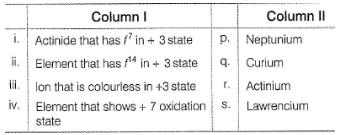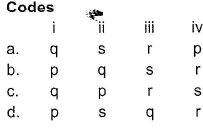Solution:

(i)→ (q), (ii) → (s), (iii) → (r) , (iv) → (p)

*Answer can only contain numeric values
QUESTION: 19

One Integer Value Correct Type

Direction (Q. Nos. 19-23) This section contains 5 questions. When worked out wiil result in an integer from 0 to 9 (both inclusive).

Q.

The number of electrons present in 5f subshell of berkelium are

Solution:

Berkelium (Z = 97) : [Rn] 5f96d07s2

*Answer can only contain numeric values
QUESTION: 20

The number of electrons present in 5f subshell of Th4+ ion are

Solution:

Thorium +4 configuration (Z = 90) = [Rn] 5f06d07s0

*Answer can only contain numeric values
QUESTION: 21

Number of elements that show + 6 oxidation state among Th, U, Np, Pu, Am, Cm, Bk, Cf, Es, Fm are

Solution:

Only U, Np, Pu and Am show +6 oxidation state.

*Answer can only contain numeric values
QUESTION: 22

The number of unpaired electrons in the element with atomic number 100 are

Solution:

The element with atomic number 100 is fermium. Its configuration is [Rn] 5f126d07s2.

*Answer can only contain numeric values
QUESTION: 23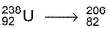Pb, in this transformation, number of alpha particles (α) liberated

Solution:

Number of alpha particles (α) liberated = change in mass number/4 = 238 - 206/4 = 8

QUESTION: 24

Statement Type

Direction (Q. Nos. 24 and 25) This section is based on Statement I and Statement II. Select the correct answer from the codes given below.

Q.

Statement I : Actinides show a large number of oxidation state whereas lanthanides show a limited number of oxidation state.

Statement Il : Energy gap between 4f, 5d and 6s subshells is small whereas that between 5f, 6d and 7s subshell is large.

Solution:

The energy gap among 4f, 5d and 6s is large where as the energy gap among 5f, 6d and 7s is small.

QUESTION: 25

Statement I : Actinides form relatively less stable complexes as compared to lanthanides.

Statement II : Actinides can utilise their 5f -orbitals along with 6d-orbitals in bonding but lanthanides do not use their 4f-orbitals for bonding.

Solution:

Acitinides form relatively more stable complexes as compared to lanthanides.